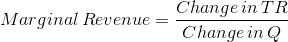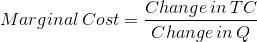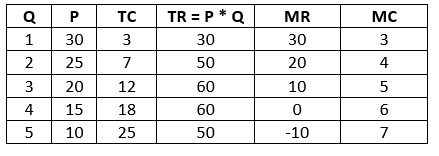# Quantity: 1 2 3 4 5. Price: $30,$25, $20,$15, $10. Total Cost:$3, $7,$12, $18,$25. 1) To...

## Question:

Use the following data on quantity, price, and total cost to answer the questions below.

Quantity: 1 2 3 4 5

Price: $30,$25, $20,$15, $10 Total Cost:$3, $7,$12, $18,$25

## Costs, revenue and profit of a monopoly.

As any other types of market structures, a monopoly wants to maximize its profit. For this, it has to produce at the level (Q) where marginal revenue is equal to marginal cost (or is as close to it as possible). To calculate the actual profit of a monopoly, one needs to find the difference between its total revenue and total cost at the optimal Q.

#### 1) To maximize profit, the monopolist sets price at _ .

To make answering all of these questions easier, we can create a table, in which P, Q and TC are given.

Everything else is calculated as following, where TR - total revenue, MR - marginal revenue, MC - marginal cost.

TR = P * QProfit is maximized at Q where MR = MC or is as close to it as possible.

Here, at no Q is MR equal to MC, but the smallest positive difference between the two is at Q = 3 ( MR - MC = 5 )

When Q = 3, P = 20.

#### 2) The marginal revenue of the second unit is _.

From the 5th column of the table, when Q = 2, MR = 20.

#### 3) The marginal cost of the fourth unit is .

From the 6th column of the table, when Q = 4, MC = 6.

#### 4) The maximum profit this monopolist can earn is .

Per part 1), the maximum profit would occur at Q = 3.

Profit = TR - TC

when Q = 3, profit = 60 - 12 = 48.